## 2.2 The Limit of a Function

### Learning Objectives

• Using correct notation, describe the limit of a function.
• Use a table of values to estimate the limit of a function or to identify when the limit does not exist.
• Use a graph to estimate the limit of a function or to identify when the limit does not exist.
• Define one-sided limits and provide examples.
• Explain the relationship between one-sided and two-sided limits.
• Using correct notation, describe an infinite limit.
• Define a vertical asymptote.

The concept of a limit or limiting process, essential to the understanding of calculus, has been around for thousands of years. In fact, early mathematicians used a limiting process to obtain better and better approximations of areas of circles. Yet, the formal definition of a limit—as we know and understand it today—did not appear until the late 19th century. We therefore begin our quest to understand limits, as our mathematical ancestors did, by using an intuitive approach. At the end of this chapter, armed with a conceptual understanding of limits, we examine the formal definition of a limit.

We begin our exploration of limits by taking a look at the graphs of the functions

$f(x)=\frac{x^2-4}{x-2}, \, g(x)=\frac{|x-2|}{x-2}$, and $h(x)=\frac{1}{(x-2)^2}$,

which are shown in (Figure). In particular, let’s focus our attention on the behavior of each graph at and around $x=2$.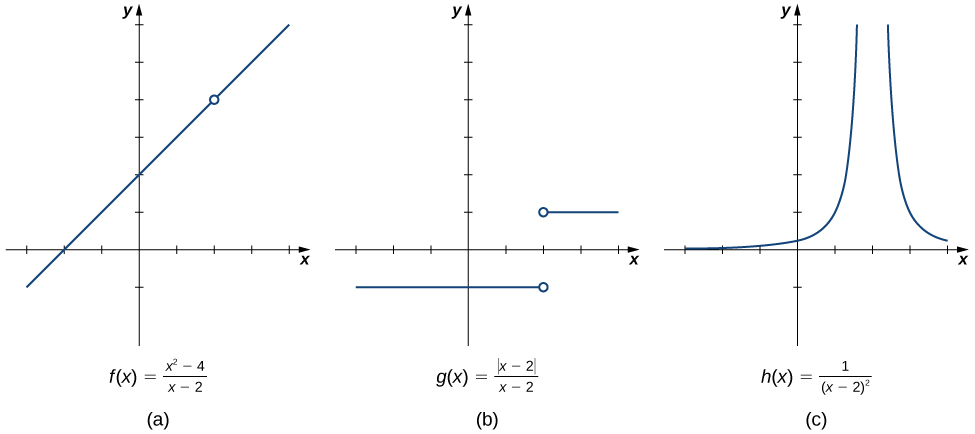Figure 1. These graphs show the behavior of three different functions around $x=2$.

Each of the three functions is undefined at $x=2$, but if we make this statement and no other, we give a very incomplete picture of how each function behaves in the vicinity of $x=2$. To express the behavior of each graph in the vicinity of 2 more completely, we need to introduce the concept of a limit.

# Intuitive Definition of a Limit

Let’s first take a closer look at how the function $f(x)=(x^2-4)/(x-2)$ behaves around $x=2$ in (Figure). As the values of $x$ approach 2 from either side of 2, the values of $y=f(x)$ approach 4. Mathematically, we say that the limit of $f(x)$ as $x$ approaches 2 is 4. Symbolically, we express this limit as

$\underset{x \to 2}{\lim}f(x)=4$.

From this very brief informal look at one limit, let’s start to develop an intuitive definition of the limit. We can think of the limit of a function at a number $a$ as being the one real number $L$ that the functional values approach as the $x$-values approach $a$, provided such a real number $L$ exists. Stated more carefully, we have the following definition:

### Definition

Let $f(x)$ be a function defined at all values in an open interval containing $a$, with the possible exception of $a$ itself, and let $L$ be a real number. If all values of the function $f(x)$ approach the real number $L$ as the values of $x(\ne a)$ approach the number $a$, then we say that the limit of $f(x)$ as $x$ approaches $a$ is $L$. (More succinct, as $x$ gets closer to $a$, $f(x)$ gets closer and stays close to $L$.) Symbolically, we express this idea as

$\underset{x\to a}{\lim}f(x)=L$.

We can estimate limits by constructing tables of functional values and by looking at their graphs. This process is described in the following Problem-Solving Strategy.

### Problem-Solving Strategy: Evaluating a Limit Using a Table of Functional Values

1. To evaluate $\underset{x\to a}{\lim}f(x)$, we begin by completing a table of functional values. We should choose two sets of $x$-values—one set of values approaching $a$ and less than $a$, and another set of values approaching $a$ and greater than $a$. (Figure) demonstrates what your tables might look like.
Table of Functional Values for $\underset{x\to a}{\lim}f(x)$
$x$ $f(x)$ $x$ $f(x)$
$a-0.1$ $f(a-0.1)$ $a+0.1$ $f(a+0.1)$
$a-0.01$ $f(a-0.01)$ $a+0.01$ $f(a+0.01)$
$a-0.001$ $f(a-0.001)$ $a+0.001$ $f(a+0.001)$
$a-0.0001$ $f(a-0.0001)$ $a+0.0001$ $f(a+0.0001)$
2. Next, let’s look at the values in each of the $f(x)$ columns and determine whether the values seem to be approaching a single value as we move down each column. In our columns, we look at the sequence $f(a-0.1), \, f(a-0.01), \, f(a-0.001), \, f(a-0.0001),$ and so on, and $f(a+0.1), \, f(a+0.01), \, f(a+0.001), \, f(a+0.0001)$ and so on. (Note: Although we have chosen the $x$-values $a \pm 0.1, \, a \pm 0.01, \, a \pm 0.001, \, a \pm 0.0001$, and so forth, and these values will probably work nearly every time, on very rare occasions we may need to modify our choices.)
3. If both columns approach a common $y$-value $L$, we state $\underset{x\to a}{\lim}f(x)=L$. We can use the following strategy to confirm the result obtained from the table or as an alternative method for estimating a limit.
4. Using a graphing calculator or computer software that allows us graph functions, we can plot the function $f(x)$, making sure the functional values of $f(x)$ for $x$-values near $a$ are in our window. We can use the trace feature to move along the graph of the function and watch the $y$-value readout as the $x$-values approach $a$. If the $y$-values approach $L$ as our $x$-values approach $a$ from both directions, then $\underset{x\to a}{\lim}f(x)=L$. We may need to zoom in on our graph and repeat this process several times.

We apply this Problem-Solving Strategy to compute a limit in (Figure).

### Evaluating a Limit Using a Table of Functional Values 1

Evaluate $\underset{x\to 0}{\lim}\frac{\sin x}{x}$ using a table of functional values.

### Evaluating a Limit Using a Table of Functional Values 2

Evaluate $\underset{x\to 4}{\lim}\frac{\sqrt{x}-2}{x-4}$ using a table of functional values.

Estimate $\underset{x\to 1}{\lim}\frac{\frac{1}{x}-1}{x-1}$ using a table of functional values. Use a graph to confirm your estimate.

#### Hint

Use 0.9, 0.99, 0.999, 0.9999, 0.99999 and 1.1, 1.01, 1.001, 1.0001, 1.00001 as your table values.

At this point, we see from (Figure) and (Figure) that it may be just as easy, if not easier, to estimate a limit of a function by inspecting its graph as it is to estimate the limit by using a table of functional values. In (Figure), we evaluate a limit exclusively by looking at a graph rather than by using a table of functional values.

### Evaluating a Limit Using a Graph

For $g(x)$ shown in (Figure), evaluate $\underset{x\to -1}{\lim}g(x)$.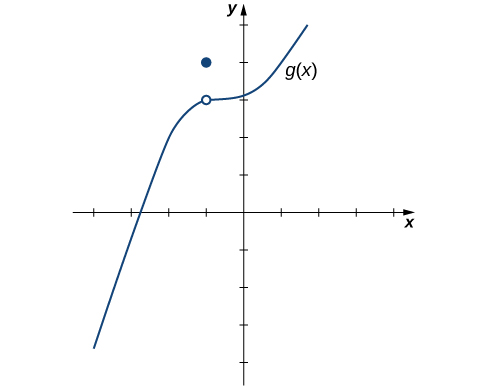Figure 4. The graph of $g(x)$ includes one value not on a smooth curve.

Based on (Figure), we make the following observation: It is possible for the limit of a function to exist at a point, and for the function to be defined at this point, but the limit of the function and the value of the function at the point may be different.

Use the graph of $h(x)$ in (Figure) to evaluate $\underset{x\to 2}{\lim}h(x)$, if possible.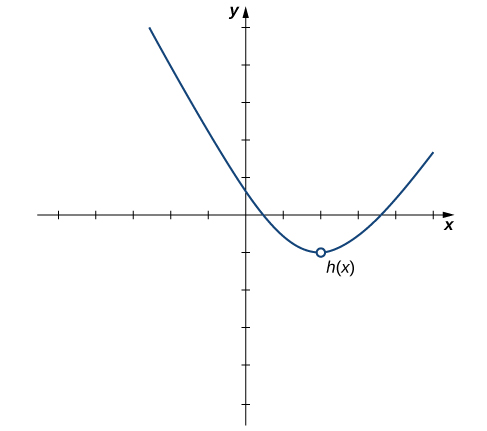Figure 5.  The graph of $h(x)$ consists of a smooth graph with a single removed point at $x=2$.

#### Hint

What $y$-value does the function approach as the $x$-values approach 2?

Looking at a table of functional values or looking at the graph of a function provides us with useful insight into the value of the limit of a function at a given point. However, these techniques rely too much on guesswork. We eventually need to develop alternative methods of evaluating limits. These new methods are more algebraic in nature and we explore them in the next section; however, at this point we introduce two special limits that are foundational to the techniques to come.

### Two Important Limits

Let $a$ be a real number and $c$ be a constant.

1. $\underset{x\to a}{\lim}x=a$
2. $\underset{x\to a}{\lim}c=c$

We can make the following observations about these two limits.

1. For the first limit, observe that as $x$ approaches $a$, so does $f(x)$, because $f(x)=x$. Consequently, $\underset{x\to a}{\lim}x=a$.
2. For the second limit, consider (Figure).
Table of Functional Values for $\underset{x\to a}{\lim}c=c$
$x$ $f(x)=c$ $x$ $f(x)=c$
$a-0.1$ $c$ $a+0.1$ $c$
$a-0.01$ $c$ $a+0.01$ $c$
$a-0.001$ $c$ $a+0.001$ $c$
$a-0.0001$ $c$ $a+0.0001$ $c$

Observe that for all values of $x$ (regardless of whether they are approaching $a$), the values $f(x)$ remain constant at $c$. We have no choice but to conclude $\underset{x\to a}{\lim}c=c$.

# The Existence of a Limit

As we consider the limit in the next example, keep in mind that for the limit of a function to exist at a point, the functional values must approach a single real-number value at that point. If the functional values do not approach a single value, then the limit does not exist.

### Evaluating a Limit That Fails to Exist

Evaluate $\underset{x\to 0}{\lim} \sin (1/x)$ using a table of values.

Use a table of functional values to evaluate $\underset{x\to 2}{\lim}\frac{|x^2-4|}{x-2}$, if possible.

#### Hint

Use $x$-values 1.9, 1.99, 1.999, 1.9999, 1.9999 and 2.1, 2.01, 2.001, 2.0001, 2.00001 in your table.

# One-Sided Limits

Sometimes indicating that the limit of a function fails to exist at a point does not provide us with enough information about the behavior of the function at that particular point. To see this, we now revisit the function $g(x)=|x-2|/(x-2)$ introduced at the beginning of the section (see (Figure)(b)). As we pick values of $x$ close to 2, $g(x)$ does not approach a single value, so the limit as $x$ approaches 2 does not exist—that is, $\underset{x\to 2}{\lim}g(x)$ DNE. However, this statement alone does not give us a complete picture of the behavior of the function around the $x$-value 2. To provide a more accurate description, we introduce the idea of a one-sided limit. For all values to the left of 2 (or the negative side of 2), $g(x)=-1$. Thus, as $x$ approaches 2 from the left, $g(x)$ approaches −1. Mathematically, we say that the limit as $x$ approaches 2 from the left is −1. Symbolically, we express this idea as

$\underset{x\to 2^-}{\lim}g(x)=-1$.

Similarly, as $x$ approaches 2 from the right (or from the positive side), $g(x)$ approaches 1. Symbolically, we express this idea as

$\underset{x\to 2^+}{\lim}g(x)=1$.

We can now present an informal definition of one-sided limits.

### Definition

We define two types of one-sided limits.

Limit from the left: Let $f(x)$ be a function defined at all values in an open interval of the form z, and let $L$ be a real number. If the values of the function $f(x)$ approach the real number $L$ as the values of $x$ (where $x<a$) approach the number $a$, then we say that $L$ is the limit of $f(x)$ as $x$ approaches a from the left. Symbolically, we express this idea as

$\underset{x\to a^-}{\lim}f(x)=L$.

Limit from the right: Let $f(x)$ be a function defined at all values in an open interval of the form $(a,c)$, and let $L$ be a real number. If the values of the function $f(x)$ approach the real number $L$ as the values of $x$ (where $x>a$) approach the number $a$, then we say that $L$ is the limit of $f(x)$ as $x$ approaches $a$ from the right. Symbolically, we express this idea as

$\underset{x\to a^+}{\lim}f(x)=L$.

### Evaluating One-Sided Limits

For the function $f(x)=\begin{cases} x+1, & \text{if} \, x < 2 \\ x^2-4, & \text{if} \, x \ge 2 \end{cases}$, evaluate each of the following limits.

1. $\underset{x\to 2^-}{\lim}f(x)$
2. $\underset{x\to 2^+}{\lim}f(x)$

Use a table of functional values to estimate the following limits, if possible.

1. $\underset{x\to 2^-}{\lim}\frac{|x^2-4|}{x-2}$
2. $\underset{x\to 2^+}{\lim}\frac{|x^2-4|}{x-2}$

#### Hint

1. Use $x$-values 1.9, 1.99, 1.999, 1.9999, 1.9999 to estimate $\underset{x\to 2^-}{\lim}\frac{|x^2-4|}{x-2}$.
2. Use $x$-values 2.1, 2.01, 2.001, 2.0001, 2.00001 to estimate $\underset{x\to 2^+}{\lim}\frac{|x^2-4|}{x-2}$.
(These tables are available from a previous Checkpoint problem.)

Let us now consider the relationship between the limit of a function at a point and the limits from the right and left at that point. It seems clear that if the limit from the right and the limit from the left have a common value, then that common value is the limit of the function at that point. Similarly, if the limit from the left and the limit from the right take on different values, the limit of the function does not exist. These conclusions are summarized in (Figure).

### Relating One-Sided and Two-Sided Limits

Let $f(x)$ be a function defined at all values in an open interval containing $a$, with the possible exception of $a$ itself, and let $L$ be a real number. Then,

$\underset{x\to a}{\lim}f(x)=L$ if and only if $\underset{x\to a^-}{\lim}f(x)=L$ and $\underset{x\to a^+}{\lim}f(x)=L$.

# Infinite Limits

Evaluating the limit of a function at a point or evaluating the limit of a function from the right and left at a point helps us to characterize the behavior of a function around a given value. As we shall see, we can also describe the behavior of functions that do not have finite limits.

We now turn our attention to $h(x)=1/(x-2)^2$, the third and final function introduced at the beginning of this section (see (Figure)(c)). From its graph we see that as the values of $x$ approach 2, the values of $h(x)=1/(x-2)^2$ become larger and larger and, in fact, become infinite. Mathematically, we say that the limit of $h(x)$ as $x$ approaches 2 is positive infinity. Symbolically, we express this idea as

$\underset{x\to 2}{\lim}h(x)=+\infty$.

More generally, we define infinite limits as follows:

### Definition

We define three types of infinite limits.

Infinite limits from the left: Let $f(x)$ be a function defined at all values in an open interval of the form $(b,a)$.

1. If the values of $f(x)$ increase without bound as the values of $x$ (where $x<a$) approach the number $a$, then we say that the limit as $x$ approaches $a$ from the left is positive infinity and we write
$\underset{x\to a^-}{\lim}f(x)=+\infty$.
2. If the values of $f(x)$ decrease without bound as the values of $x$ (where $x<a$) approach the number $a$, then we say that the limit as $x$ approaches $a$ from the left is negative infinity and we write
$\underset{x\to a^-}{\lim}f(x)=−\infty$.

Infinite limits from the right: Let $f(x)$ be a function defined at all values in an open interval of the form $(a,c)$.

1. If the values of $f(x)$ increase without bound as the values of $x$ (where $x>a$) approach the number $a$, then we say that the limit as $x$ approaches $a$ from the left is positive infinity and we write
$\underset{x\to a^+}{\lim}f(x)=+\infty$.
2. If the values of $f(x)$ decrease without bound as the values of $x$ (where $x>a$) approach the number $a$, then we say that the limit as $x$ approaches $a$ from the left is negative infinity and we write
$\underset{x\to a^+}{\lim}f(x)=−\infty$.

Two-sided infinite limit: Let $f(x)$ be defined for all $x\ne a$ in an open interval containing $a$.

1. If the values of $f(x)$ increase without bound as the values of $x$ (where $x\ne a$) approach the number $a$, then we say that the limit as $x$ approaches $a$ is positive infinity and we write
$\underset{x\to a}{\lim}f(x)=+\infty$.
2. If the values of $f(x)$ decrease without bound as the values of $x$ (where $x\ne a$) approach the number $a$, then we say that the limit as $x$ approaches $a$ is negative infinity and we write
$\underset{x\to a}{\lim}f(x)=−\infty$.

It is important to understand that when we write statements such as $\underset{x\to a}{\lim}f(x)=+\infty$ or $\underset{x\to a}{\lim}f(x)=−\infty$ we are describing the behavior of the function, as we have just defined it. We are not asserting that a limit exists. For the limit of a function $f(x)$ to exist at $a$, it must approach a real number $L$ as $x$ approaches $a$. That said, if, for example, $\underset{x\to a}{\lim}f(x)=+\infty$, we always write $\underset{x\to a}{\lim}f(x)=+\infty$ rather than $\underset{x\to a}{\lim}f(x)$ DNE.

### Recognizing an Infinite Limit

Evaluate each of the following limits, if possible. Use a table of functional values and graph $f(x)=1/x$ to confirm your conclusion.

1. $\underset{x\to 0^-}{\lim}\frac{1}{x}$
2. $\underset{x\to 0^+}{\lim}\frac{1}{x}$
3. $\underset{x\to 0}{\lim}\frac{1}{x}$

Evaluate each of the following limits, if possible. Use a table of functional values and graph $f(x)=1/x^2$ to confirm your conclusion.

1. $\underset{x\to 0^-}{\lim}\frac{1}{x^2}$
2. $\underset{x\to 0^+}{\lim}\frac{1}{x^2}$
3. $\underset{x\to 0}{\lim}\frac{1}{x^2}$

#### Hint

It is useful to point out that functions of the form $f(x)=1/(x-a)^n$, where $n$ is a positive integer, have infinite limits as $x$ approaches $a$ from either the left or right ((Figure)). These limits are summarized in (Figure).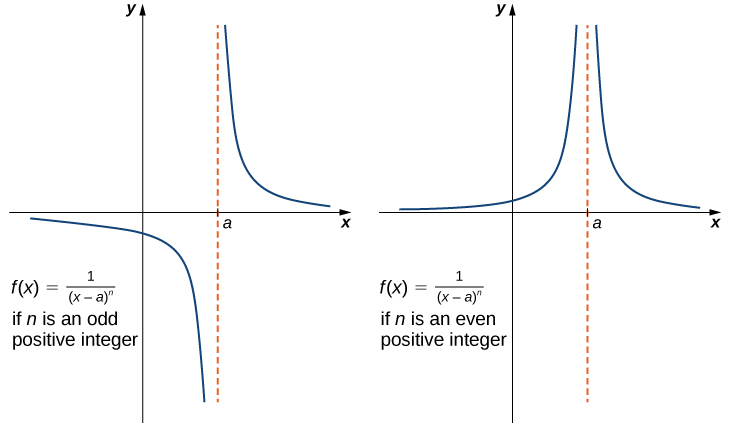Figure 9. The function $f(x)=1/(x-a)^n$ has infinite limits at $a$.

### Infinite Limits from Positive Integers

If $n$ is a positive even integer, then

$\underset{x\to a}{\lim}\frac{1}{(x-a)^n}=+\infty$.

If $n$ is a positive odd integer, then

$\underset{x\to a^+}{\lim}\frac{1}{(x-a)^n}=+\infty$

and

$\underset{x\to a^-}{\lim}\frac{1}{(x-a)^n}=−\infty$.

We should also point out that in the graphs of $f(x)=1/(x-a)^n$, points on the graph having $x$-coordinates very near to $a$ are very close to the vertical line $x=a$. That is, as $x$ approaches $a$, the points on the graph of $f(x)$ are closer to the line $x=a$. The line $x=a$ is called a vertical asymptote of the graph. We formally define a vertical asymptote as follows:

### Definition

Let $f(x)$ be a function. If any of the following conditions hold, then the line $x=a$ is a vertical asymptote of $f(x)$:

$\begin{array}{ccc}\hfill \underset{x\to a^-}{\lim}f(x)& =\hfill & +\infty \, \text{or} \, -\infty \hfill \\ \hfill \underset{x\to a^+}{\lim}f(x)& =\hfill & +\infty \, \text{or} \, −\infty \hfill \\ & \text{or}\hfill & \\ \hfill \underset{x\to a}{\lim}f(x)& =\hfill & +\infty \, \text{or} \, −\infty \hfill \end{array}$

### Finding a Vertical Asymptote

Evaluate each of the following limits using (Figure). Identify any vertical asymptotes of the function $f(x)=1/(x+3)^4$.

1. $\underset{x\to -3^-}{\lim}\frac{1}{(x+3)^4}$
2. $\underset{x\to -3^+}{\lim}\frac{1}{(x+3)^4}$
3. $\underset{x\to -3}{\lim}\frac{1}{(x+3)^4}$

Evaluate each of the following limits. Identify any vertical asymptotes of the function $f(x)=\frac{1}{(x-2)^3}$.

1. $\underset{x\to 2^-}{\lim}\frac{1}{(x-2)^3}$
2. $\underset{x\to 2^+}{\lim}\frac{1}{(x-2)^3}$
3. $\underset{x\to 2}{\lim}\frac{1}{(x-2)^3}$

#### Hint

Use (Figure).

In the next example we put our knowledge of various types of limits to use to analyze the behavior of a function at several different points.

### Behavior of a Function at Different Points

Use the graph of $f(x)$ in (Figure) to determine each of the following values:

1. $\underset{x\to -4^-}{\lim}f(x); \, \underset{x\to -4^+}{\lim}f(x); \, \underset{x\to -4}{\lim}f(x); \, f(-4)$
2. $\underset{x\to -2^-}{\lim}f(x); \, \underset{x\to -2^+}{\lim}f(x); \, \underset{x\to -2}{\lim}f(x); \, f(-2)$
3. $\underset{x\to 1^-}{\lim}f(x); \, \underset{x\to 1^+}{\lim}f(x); \, \underset{x\to 1}{\lim}f(x); \, f(1)$
4. $\underset{x\to 3^-}{\lim}f(x); \, \underset{x\to 3^+}{\lim}f(x); \, \underset{x\to 3}{\lim}f(x); \, f(3)$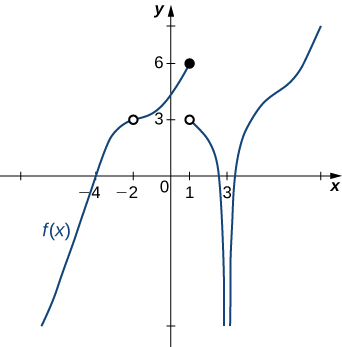Figure 10. The graph shows $f(x)$.

Evaluate $\underset{x\to 1}{\lim}f(x)$ for $f(x)$ shown here: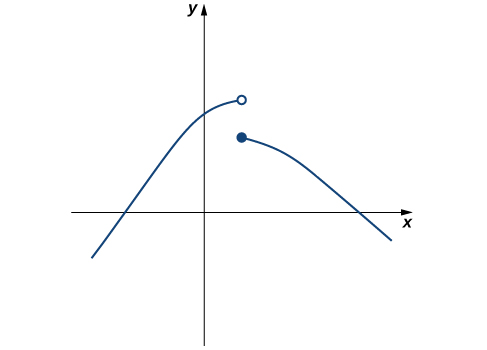#### Hint

Compare the limit from the right with the limit from the left.

### Chapter Opener: Einstein’s Equation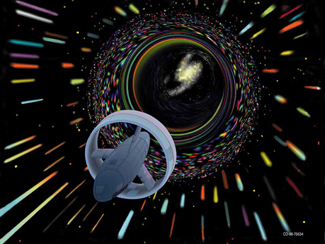Figure 11. (credit: NASA)

In the chapter opener we mentioned briefly how Albert Einstein showed that a limit exists to how fast any object can travel. Given Einstein’s equation for the mass of a moving object, what is the value of this bound?

### Key Concepts

• A table of values or graph may be used to estimate a limit.
• If the limit of a function at a point does not exist, it is still possible that the limits from the left and right at that point may exist.
• If the limits of a function from the left and right exist and are equal, then the limit of the function is that common value.
• We may use limits to describe infinite behavior of a function at a point.

# Key Equations

• Intuitive Definition of the Limit
$\underset{x\to a}{\lim}f(x)=L$
• Two Important Limits
$\underset{x\to a}{\lim}x=a$
$\underset{x\to a}{\lim}c=c$
• One-Sided Limits
$\underset{x\to a^-}{\lim}f(x)=L$
$\underset{x\to a^+}{\lim}f(x)=L$
• Infinite Limits from the Left
$\underset{x\to a^-}{\lim}f(x)=+\infty$
$\underset{x\to a^-}{\lim}f(x)=−\infty$
• Infinite Limits from the Right
$\underset{x\to a^+}{\lim}f(x)=+\infty$
$\underset{x\to a^+}{\lim}f(x)=−\infty$
• Two-Sided Infinite Limits
$\underset{x\to a}{\lim}f(x)=+\infty: \underset{x\to a^-}{\lim}f(x)=+\infty$ and $\underset{x\to a^+}{\lim}f(x)=+\infty$
$\underset{x\to a}{\lim}f(x)=−\infty: \underset{x\to a^-}{\lim}f(x)=−\infty$ and $\underset{x\to a^+}{\lim}f(x)=−\infty$

For the following exercises, consider the function $f(x)=\frac{x^2-1}{|x-1|}$.

1. [T] Complete the following table for the function. Round your solutions to four decimal places.

$x$ $f(x)$ $x$ $f(x)$
0.9 a. 1.1 e.
0.99 b. 1.01 f.
0.999 c. 1.001 g.
0.9999 d. 1.0001 h.

2. What do your results in the preceding exercise indicate about the two-sided limit $\underset{x\to 1}{\lim}f(x)$? Explain your response.

For the following exercises, consider the function $f(x)=(1+x)^{1/x}$.

3. [T] Make a table showing the values of $f$ for $x=-0.01, \, -0.001, \, -0.0001, \, -0.00001$ and for $x=0.01, \, 0.001, \, 0.0001, \, 0.00001$. Round your solutions to five decimal places.

$x$ $f(x)$ $x$ $f(x)$
−0.01 a. 0.01 e.
−0.001 b. 0.001 f.
−0.0001 c. 0.0001 g.
−0.00001 d. 0.00001 h.

4. What does the table of values in the preceding exercise indicate about the function $f(x)=(1+x)^{1/x}$?

5. To which mathematical constant does the limit in the preceding exercise appear to be getting closer?

In the following exercises, use the given values of $x$ to set up a table to evaluate the limits. Round your solutions to eight decimal places.

6. [T]$\underset{x\to 0}{\lim}\frac{\sin 2x}{x}; \, x = \pm 0.1, \, \pm 0.01, \, \pm 0.001, \, \pm 0.0001$

$x$ $\frac{\sin 2x}{x}$ $x$ $\frac{\sin 2x}{x}$
−0.1 a. 0.1 e.
−0.01 b. 0.01 f.
−0.001 c. 0.001 g.
−0.0001 d. 0.0001 h.

7. [T]$\underset{x\to 0}{\lim}\frac{\sin 3x}{x}; \, x = \pm 0.1, \, \pm 0.01, \, \pm 0.001, \, \pm 0.0001$

X $\frac{\sin 3x}{x}$ $x$ $\frac{\sin 3x}{x}$
−0.1 a. 0.1 e.
−0.01 b. 0.01 f.
−0.001 c. 0.001 g.
−0.0001 d. 0.0001 h.

8. Use the preceding two exercises to conjecture (guess) the value of the following limit: $\underset{x\to 0}{\lim}\frac{\sin ax}{x}$ for $a$, a positive real value.

In the following exercises, set up a table of values to find the indicated limit. Round to eight digits.

9. [T] $\underset{x\to 2}{\lim}\frac{x^2-4}{x^2+x-6}$

$x$ $\frac{x^2-4}{x^2+x-6}$ $x$ $\frac{x^2-4}{x^2+x-6}$
1.9 a. 2.1 e.
1.99 b. 2.01 f.
1.999 c. 2.001 g.
1.9999 d. 2.0001 h.

10. [T] $\underset{x\to 1}{\lim}(1-2x)$

$x$ $1-2x$ $x$ $1-2x$
0.9 a. 1.1 e.
0.99 b. 1.01 f.
0.999 c. 1.001 g.
0.9999 d. 1.0001 h.

11. [T] $\underset{x\to 0}{\lim}\frac{5}{1-e^{1/x}}$

$x$ $\frac{5}{1-e^{1/x}}$ $x$ $\frac{5}{1-e^{1/x}}$
−0.1 a. 0.1 e.
−0.01 b. 0.01 f.
−0.001 c. 0.001 g.
−0.0001 d. 0.0001 h.

12. [T] $\underset{z\to 0}{\lim}\frac{z-1}{z^2(z+3)}$

$z$ $\frac{z-1}{z^2(z+3)}$ $z$ $\frac{z-1}{z^2(z+3)}$
−0.1 a. 0.1 e.
−0.01 b. 0.01 f.
−0.001 c. 0.001 g.
−0.0001 d. 0.0001 h.

13. [T] $\underset{t\to 0^+}{\lim}\frac{\cos t}{t}$

$t$ $\frac{\cos t}{t}$
0.1 a.
0.01 b.
0.001 c.
0.0001 d.

14. [T] $\underset{x\to 2}{\lim}\frac{1-\frac{2}{x}}{x^2-4}$

$x$ $\frac{1-\frac{2}{x}}{x^2-4}$ $x$ $\frac{1-\frac{2}{x}}{x^2-4}$
1.9 a. 2.1 e.
1.99 b. 2.01 f.
1.999 c. 2.001 g.
1.9999 d. 2.0001 h.

In the following exercises, set up a table of values and round to eight significant digits. Based on the table of values, make a guess about what the limit is. Then, use a calculator to graph the function and determine the limit. Was the conjecture correct? If not, why does the method of tables fail?

15. [T] $\underset{\theta \to 0}{\lim}\sin (\frac{\pi }{\theta })$

θ $\sin (\frac{\pi }{\theta })$ θ $\sin (\frac{\pi }{\theta })$
−0.1 a. 0.1 e.
−0.01 b. 0.01 f.
−0.001 c. 0.001 g.
−0.0001 d. 0.0001 h.

16. [T] $\underset{\alpha \to 0^+}{\lim}\frac{1}{\alpha } \cos (\frac{\pi }{\alpha })$

$a$ $\frac{1}{\alpha } \cos (\frac{\pi }{\alpha })$
0.1 a.
0.01 b.
0.001 c.
0.0001 d.

In the following exercises, consider the graph of the function $y=f(x)$ shown here. Which of the statements about $y=f(x)$ are true and which are false? Explain why a statement is false.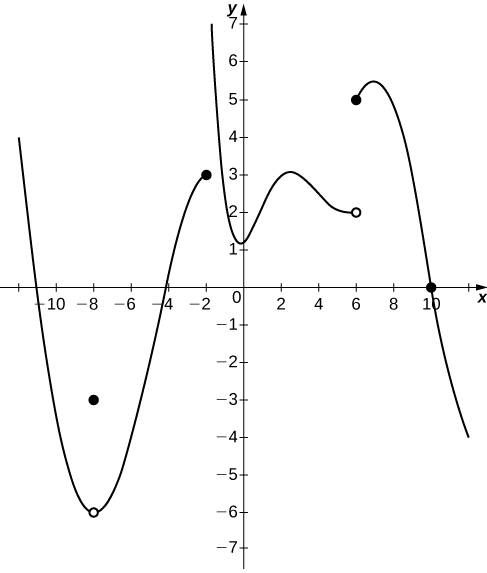17. $\underset{x\to 10}{\lim}f(x)=0$

18. $\underset{x\to -2^+}{\lim}f(x)=3$

19. $\underset{x\to -8}{\lim}f(x)=f(-8)$

20. $\underset{x\to 6}{\lim}f(x)=5$

In the following exercises, use the following graph of the function $y=f(x)$ to find the values, if possible. Estimate when necessary.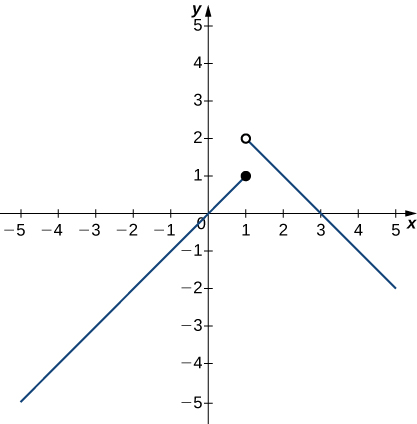21. $\underset{x\to 1^-}{\lim}f(x)$

22. $\underset{x\to 1^+}{\lim}f(x)$

23. $\underset{x\to 1}{\lim}f(x)$

24. $\underset{x\to 2}{\lim}f(x)$

25. $f(1)$

In the following exercises, use the graph of the function $y=f(x)$ shown here to find the values, if possible. Estimate when necessary.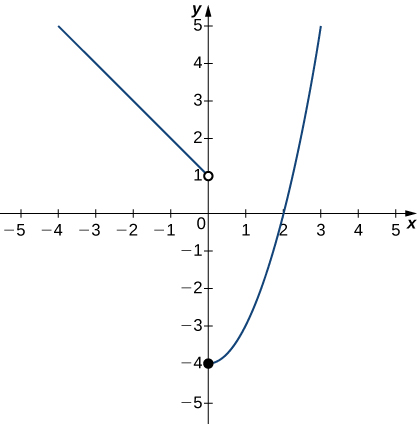26. $\underset{x\to 0^-}{\lim}f(x)$

27. $\underset{x\to 0^+}{\lim}f(x)$

28. $\underset{x\to 0}{\lim}f(x)$

29. $\underset{x\to 2}{\lim}f(x)$

In the following exercises, use the graph of the function $y=f(x)$ shown here to find the values, if possible. Estimate when necessary.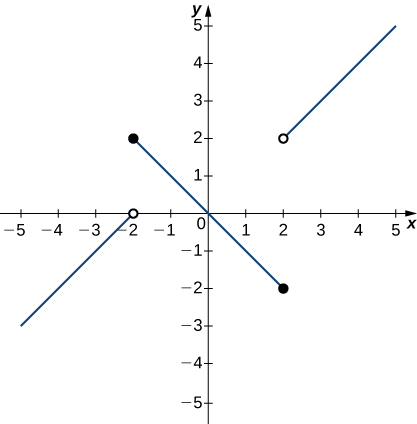30. $\underset{x\to -2^-}{\lim}f(x)$

31. $\underset{x\to -2^+}{\lim}f(x)$

32. $\underset{x\to -2}{\lim}f(x)$

33. $\underset{x\to 2^-}{\lim}f(x)$

34. $\underset{x\to 2^+}{\lim}f(x)$

35. $\underset{x\to 2}{\lim}f(x)$

In the following exercises, use the graph of the function $y=g(x)$ shown here to find the values, if possible. Estimate when necessary.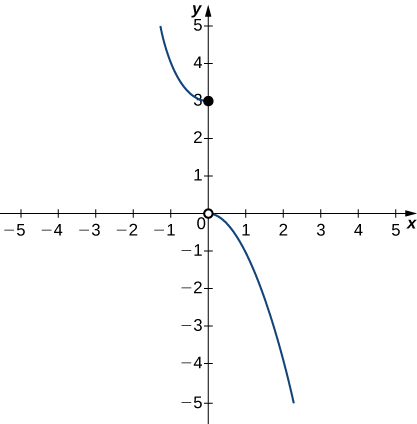36. $\underset{x\to 0^-}{\lim}g(x)$

37. $\underset{x\to 0^+}{\lim}g(x)$

38. $\underset{x\to 0}{\lim}g(x)$

In the following exercises, use the graph of the function $y=h(x)$ shown here to find the values, if possible. Estimate when necessary.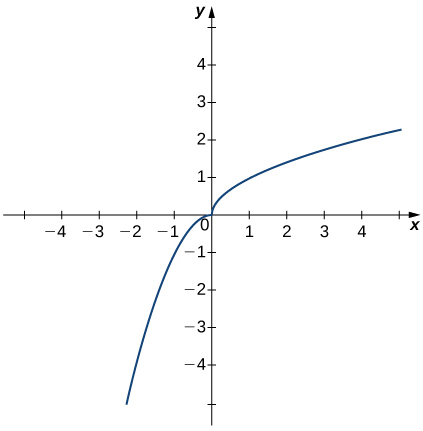39. $\underset{x\to 0^-}{\lim}h(x)$

40. $\underset{x\to 0^+}{\lim}h(x)$

41. $\underset{x\to 0}{\lim}h(x)$

In the following exercises, use the graph of the function $y=f(x)$ shown here to find the values, if possible. Estimate when necessary.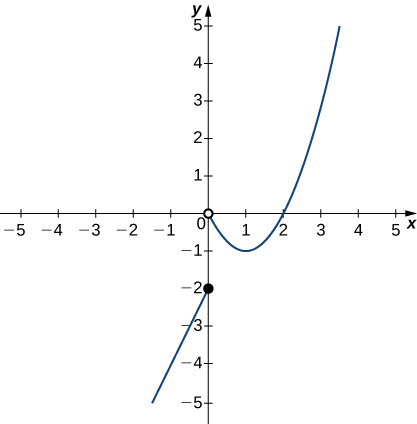42. $\underset{x\to 0^-}{\lim}f(x)$

43. $\underset{x\to 0^+}{\lim}f(x)$

44. $\underset{x\to 0}{\lim}f(x)$

45. $\underset{x\to 1}{\lim}f(x)$

46. $\underset{x\to 2}{\lim}f(x)$

In the following exercises, sketch the graph of a function with the given properties.

47. $\underset{x\to 2}{\lim}f(x)=1, \, \underset{x\to 4^-}{\lim}f(x)=3, \, \underset{x\to 4^+}{\lim}f(x)=6$, the function is not defined at $x=4$.

48. $\underset{x\to -\infty }{\lim}f(x)=0, \, \underset{x\to -1^-}{\lim}f(x)=−\infty$, $\underset{x\to -1^+}{\lim}f(x)=\infty, \, \underset{x\to 0}{\lim}f(x)=f(0), \, f(0)=1, \, \underset{x\to \infty }{\lim}f(x)=−\infty$

49. $\underset{x\to -\infty}{\lim}f(x)=2, \, \underset{x\to 3^-}{\lim}f(x)=−\infty$, $\underset{x\to 3^+}{\lim}f(x)=\infty, \, \underset{x\to \infty }{\lim}f(x)=2, \, f(0)=\frac{-1}{3}$

50. $\underset{x\to -\infty }{\lim}f(x)=2, \, \underset{x\to -2}{\lim}f(x)=−\infty$,$\underset{x\to \infty }{\lim}f(x)=2, \, f(0)=0$

51. $\underset{x\to -\infty }{\lim}f(x)=0, \, \underset{x\to -1^-}{\lim}f(x)=\infty, \, \underset{x\to -1^+}{\lim}f(x)=−\infty, \, f(0)=-1, \, \underset{x\to 1^-}{\lim}f(x)=−\infty, \, \underset{x\to 1^+}{\lim}f(x)=\infty, \, \underset{x\to \infty }{\lim}f(x)=0$

52. Shock waves arise in many physical applications, ranging from supernovas to detonation waves. A graph of the density of a shock wave with respect to distance, $x$, is shown here. We are mainly interested in the location of the front of the shock, labeled $x_\text{SF}$ in the diagram.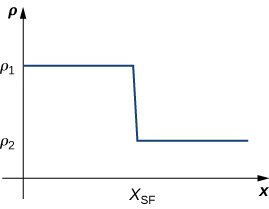1. Evaluate $\underset{x\to x_\text{SF}^+}{\lim}\rho (x)$.
2. Evaluate $\underset{x\to x_\text{SF}^-}{\lim}\rho (x)$.
3. Evaluate $\underset{x\to x_\text{SF}}{\lim}\rho (x)$. Explain the physical meanings behind your answers.

53. A track coach uses a camera with a fast shutter to estimate the position of a runner with respect to time. A table of the values of position of the athlete versus time is given here, where $x$ is the position in meters of the runner and $t$ is time in seconds. What is $\underset{t\to 2}{\lim}x(t)$? What does it mean physically?

$t$(sec) $x$(m)
1.75 4.5
1.95 6.1
1.99 6.42
2.01 6.58
2.05 6.9
2.25 8.5

## Glossary

infinite limit
A function has an infinite limit at a point $a$ if it either increases or decreases without bound as it approaches $a$
intuitive definition of the limit
If all values of the function $f(x)$ approach the real number $L$ as the values of $x(\ne a)$ approach $a$, $f(x)$ approaches $L$
one-sided limit
A one-sided limit of a function is a limit taken from either the left or the right
vertical asymptote
A function has a vertical asymptote at $x=a$ if the limit as $x$ approaches $a$ from the right or left is infinite